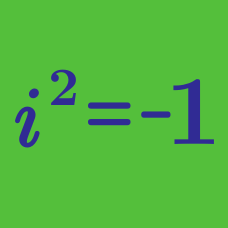Algebra

# Complex Numbers: Level 4 Challenges

$\large \begin{cases} {a_n=a_{n-1}a_{n-2}-b_{n-1}b_{n-2} } \\ { b_n=b_{n-1}a_{n-2}+a_{n-1}b_{n-2} } \end{cases}$

Two sequences $a_n$ and $b_n$ begin with values $a_1=b_1=a_2=b_2=1$ and satisfy the recursive relations above for $n>2$.

Find $\large \frac{b_{2015}}{a_{2015}}$.

How many complex numbers $z$ are there such that $|z| = 1$ and $\large{\left|\dfrac{z}{\bar{z}}+\dfrac{\bar{z}}{z}\right|} =1?$

Let $z=a+bi$, $|z|=5$, and $b>0$. If the distance between $(1+2i)z^3$ and $z^5$ is as large as possible, then determine $z^4$. Give your answer as the sum of the real and imaginary parts of $z^4$.

If $Z$ is a non-zero complex number, then find the minimum value of

$\displaystyle \dfrac{\text{Im}(Z^{5})}{(\text{Im}(Z))^{5} }.$

Clarification: $\text{Im}(Z)$ represent the imaginary part of $Z$.

For a complex number $z$, find the smallest possible value of $|z-3|^2+|z-5+2i|^2+|z-1+i|^2.$

Clarification: $i=\sqrt{-1}$.

×